# Community

Blog Events Webinars Tutorials Forum
×
Community Blog Bringing PostGIS to PostgreSQL on Alibaba Cloud (Part 2)

# Bringing PostGIS to PostgreSQL on Alibaba Cloud (Part 2)

In this part of a two part tutorial series, you learn how to perform both spatial and non-spatial function queries on ApsaraDB for PostgreSQL.

By Grace Amondi, Alibaba Cloud Community Blog author.

In this second part of this two-part tutorial series, you're going to be performing both spatial and non-spatial function queries on Alibaba Cloud ApsaraDB for PostgreSQL you had populated in the first part of this series. If you haven't yet, you should check out the part one of this series to learn how before you proceed to the steps covered in this part. For this part of the tutorial, we will be using PostGIS preview that you first dealt with in part one.

With that said, one important concept to grasp in this series is the idea of Spatial data sets, which can be primarily defined as those data sets that are directly or indirectly referencing a location on the surface of the earth. Naturally following this, when a data set is related to or is referencing a location on the surface of the earth, it is referred as non spatial data.

## Prerequisites

For you to be able to complete this tutorial, you'll need to have the following items:

1. A valid Alibaba Cloud account. If you don't have one already, sign up to a Free Trial to enjoy up to \$300 worth in Alibaba Cloud products free of charge.
2. You have an ECS instance that is installed with Ubuntu 16.04. You can select your preferred region and configurations. Your choices will not affect the outcome of the server setup.
4. You have an ApsaraDB RDS for PostgreSQL that is connected to your Ubuntu 16.04 instance.

## Procedure

To perform both spatial and non-spatial function queries on ApsaraDB for PostgreSQL, complete the steps outlined in the following sections:

### Non-Spatial Queries

With our database populated, we will now develop our very first query. Before we can start applying spatial functions, it is important that we develop knowledge of using basic standard functions on PostgreSQL.

Let's work on the places table we had created. We'll see how many places qualify as towns. Apply this simple query:

``SELECT COUNT(*),* FROM places WHERE fclass='town' GROUP BY places.gid``

You should have output that is something similar to this: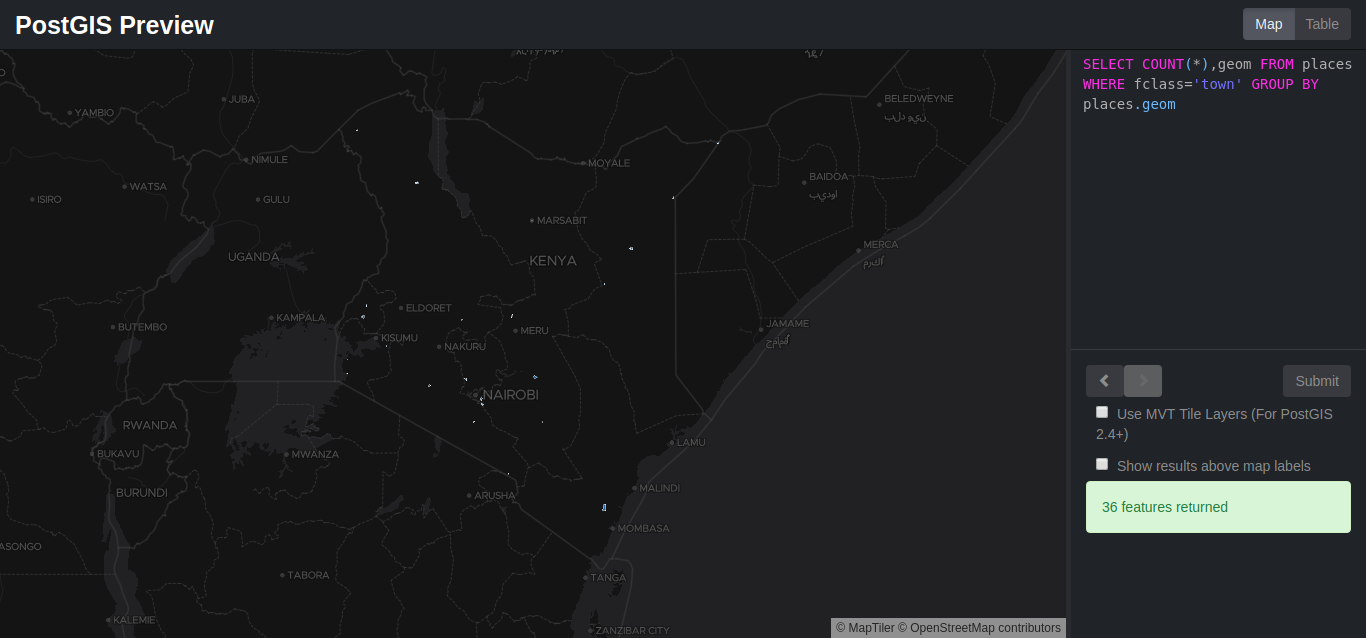And also a table like this: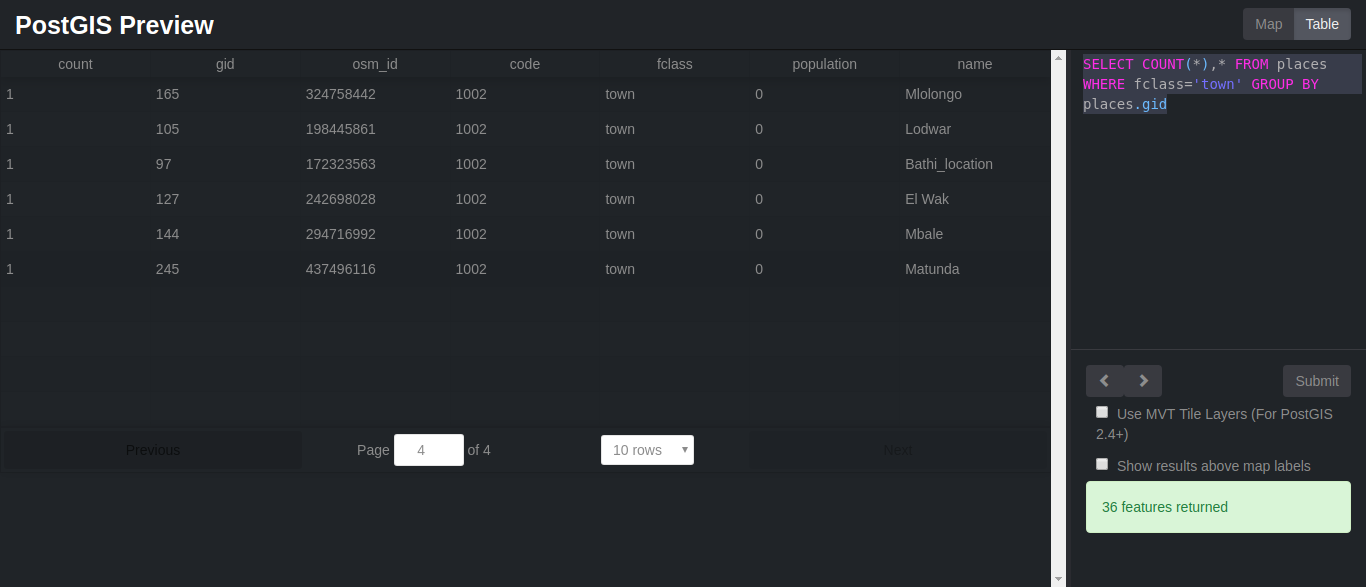Note: If it doesn't work the first time, try reloading the page. We might also want to know how many places with a specific name exist. In our example we will check how many towns by the name bamba location actually exist:

``````SELECT name, fclass, COUNT(fclass) FROM places
WHERE name = 'bamba location' AND fclass = 'town'
GROUP BY name, fclass
ORDER BY name, fclass``````

You should get a similar result of only one town. We will not be going deeper into regular queries as we need to put PostGIS to the test and perform spatial queries

### Spatial Functions

We will cover a few spatial functions and see how we can use them to extract data from our database. They are:

• ST_Distance
• ST_DWithin
• ST_Length
• ST_Intersects
• ST_Within

#### 1. The ST_Distance functions (geometry, geometry)

For geometry type returns the minimum 2D Cartesian distance between two geometries in projected units (spatial ref units). For geography type defaults to return the minimum geodesic distance between two geographies in meters. For example, POINT(-1.344165 51.381320) position is given in degrees. Another geographic reference system could use a different unit of measurement, such as meters or feet.

If we want to convert it from degrees to meters we will have to take the following into consideration:

• One degree comprises of 60 nautical miles
• 1 nautical mile is equivalent to 1852meters

Let's see how this works. We will find the distance between Kisian and Kajiado in degrees. Copy and paste the following query to postGIS preview:

``SELECT ST_DISTANCE(p1.geom, p2.geom) as distance FROM places p1, places p2 WHERE p1.name = 'Kisian' and p2.name = 'Kajiado'``

You should have something similar to the image below: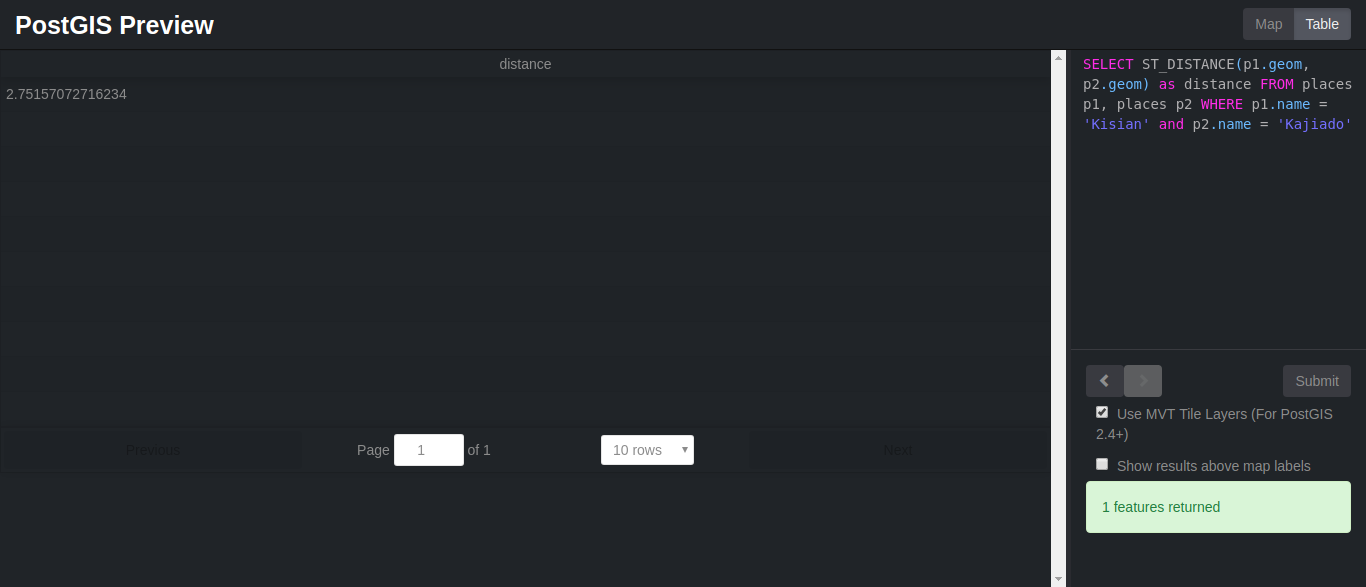Now let's try and convert the distance to meters. Type the following:

``SELECT ST_DISTANCE(p1.geom, p2.geom) as distance * 60 * 1852  FROM places p1, places p2 WHERE p1.name = 'Kisian' and p2.name = 'Kajiado'``

It should now have changed to `305754.539202279 meters`.

#### 2. The ST_DWithin function (geometry, geometry, float)

For geometry: The distance is specified in units defined by the spatial reference system of the geometries. For this function to make sense, the source geometries must both be of the same coordinate projection, having the same SRID.

For geography units are in meters and measurement is defaulted to `use_spheroid=true`, for faster check, `use_spheroid=false` to measure along sphere.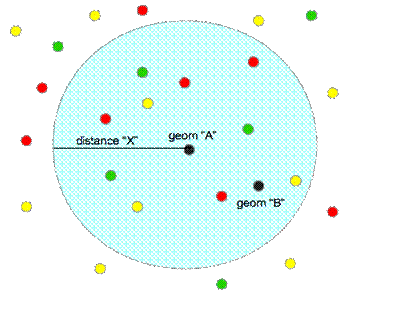Let's see how it works:

``SELECT *,h.name AS town, ST_DISTANCE(s.geom, h.geom) *69 AS distance FROM places AS h INNER JOIN places AS s ON (ST_DWITHIN(s.geom, h.geom, (1.00))) WHERE h.fclass = 'town' and s.name = 'Kajiado'``

You should have something similar to this: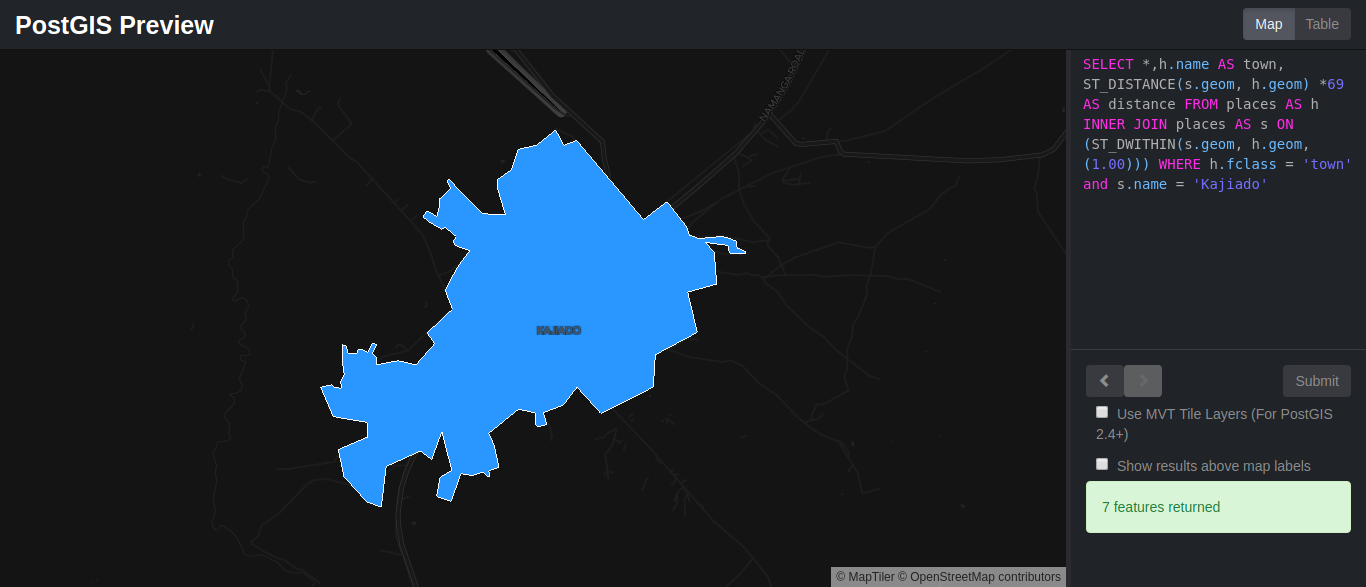And a table similar to this: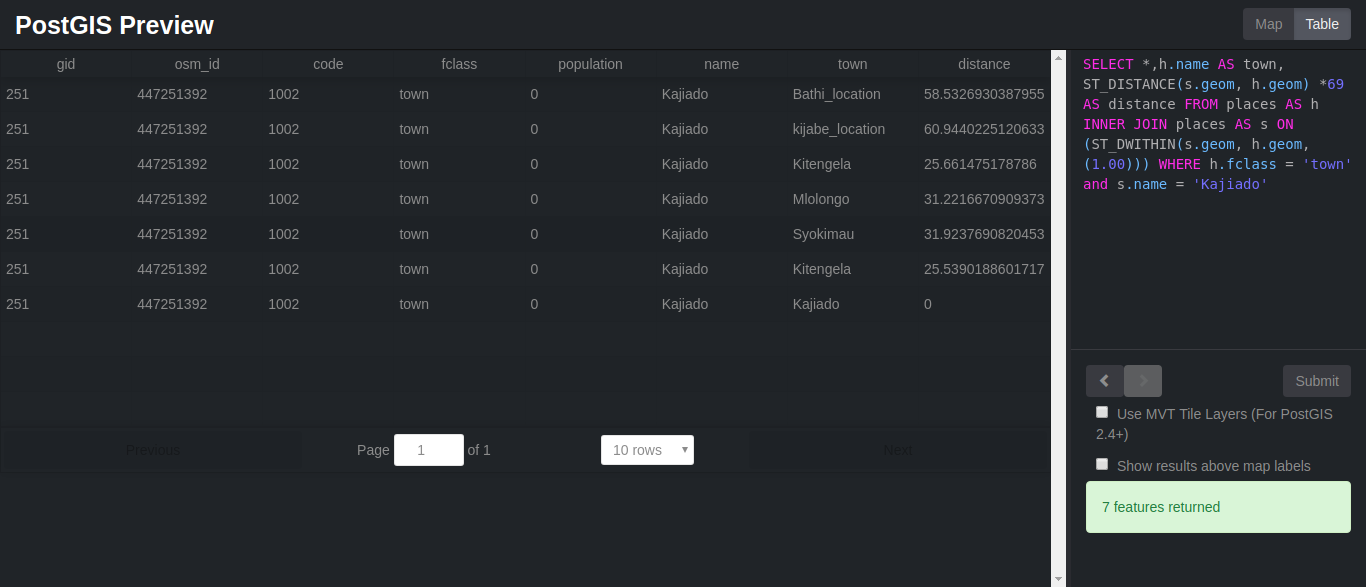#### 3. The ST_Intersects function (geometry, geometry)

If a geometry or geography shares any portion of space, then they intersect. If this returns true, then the geometries also spatially intersect. Disjoint implies false for spatial intersection.

As an example we will check on the polygons that intersect with Nairobi, if any using the query below:

``select *,ri.name FROM places AS rk INNER JOIN places AS ri ON (st_intersects(rk.geom,ri.geom)) WHERE rk.name = 'Nairobi'``

You should now be seeing something similar to this: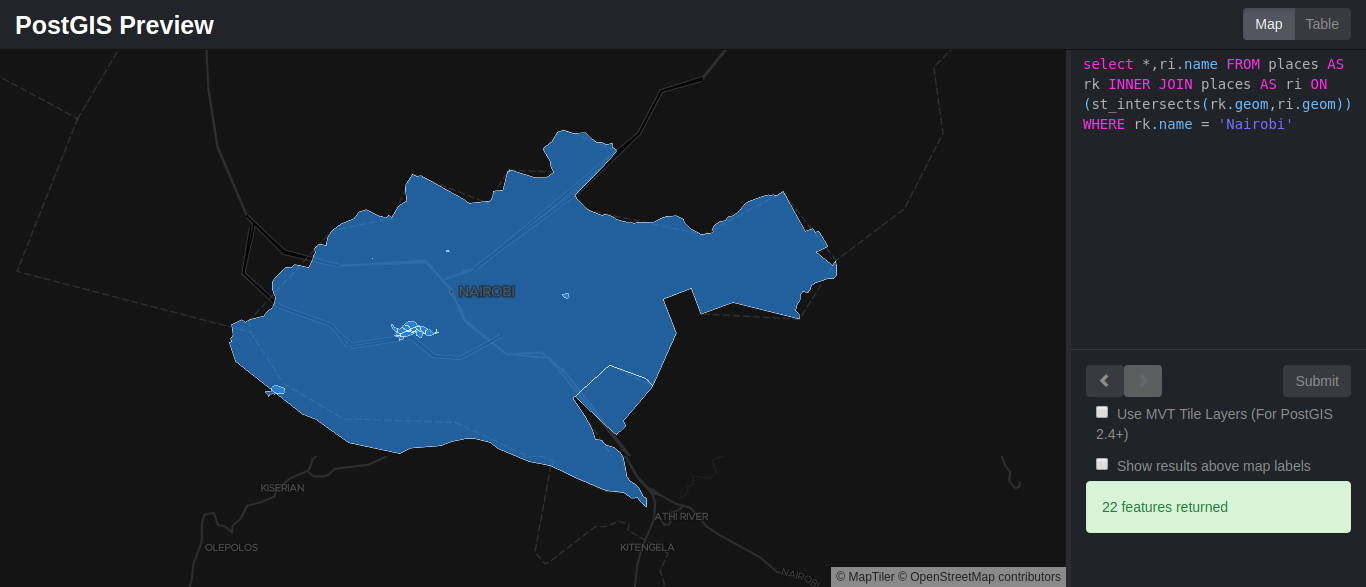#### 4. The ST_Within function (geometry A, geometry B)

The ST_Within spatial function simply returns true when Geometry A is completely inside B. Enter the query below:

``````SELECT * FROM places
WHERE ST_Within(geom, ST_GeomFromText('Polygon((9
-4.850154078505659 33.6181640625,
-4.850154078505659 42.1875,
4.806364708499998 42.1875,
4.806364708499998 33.6181640625,
-4.850154078505659 33.6181640625))',
4326))
ORDER BY name;``````

#### 5. The ST_Length function

The ST_Length function returns the length of a geometrical object if it's a line. The measurement is in the units of its spatial reference. In order to understand how it works we will try out an example.

Since we have no line geometry features, we will need to import them into our database. We will be using ogr2ogr, which is a ogr command line utility that converts one Ogr defined data source to another Ogr data source.

Using this simple command, beginning with the geojson file which we will name waterways:

``\$ ogr2ogr -f geoJSON waterways.geojson gis_osm_waterways_free_1_1.shp``

### Load GeoJSON data to Database using ogr2ogr

We can also use ogr2ogr to load the geojson file to the database directly. All we need to do is input the correct database name, the geojson file and also the table we will be creating.

Since we already have a table named waterways let's name this waterways and load the data by running the following command:

``ogr2ogr -append -f "PostgreSQL" PG:"dbname=mydatabase" waterways.geojson -nln waterways``

### Rename the geom column

At the moment the geom column is named as wkb_geometry. We need to change it to geom in relation to PostGIS PrevieLoad GeoJSON data to Database using ogr2ogrw, which only accepts the geom column as geom.

``````sh
ALTER TABLE waterways
RENAME COLUMN wkb_geometry to geom;``````

Now we can perform st_length spatial function to find the length of Likoni river by running the following query:

``````SELECT name, (ST_Length(geom))*60*1852 AS length FROM waterways
WHERE name = 'Likoni River' AND fclass ='river'``````

The table preview should look something like this: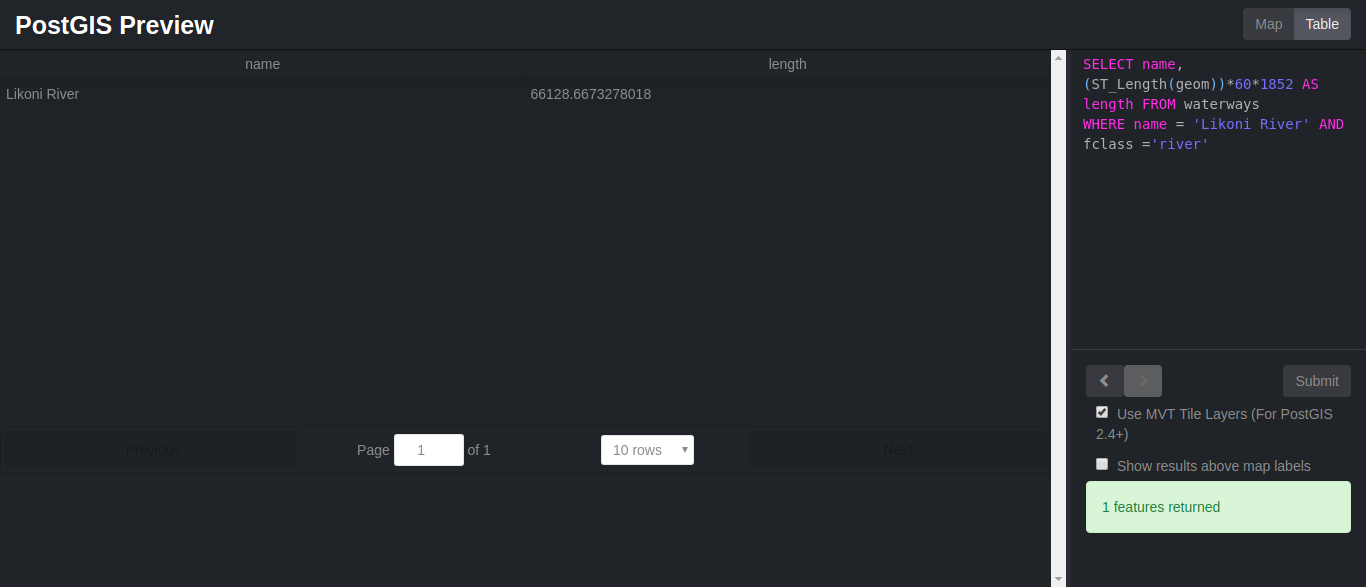There are other countless spatial functions that we have not gone through. You can check them out at PostGIS Documentation.

A bonus would be to learn how to load and generate rasters. As discussed in part 1, raster data model represents the world phenomena as cells of predefined, grid-shaped tessellation.

You could load existing raster files using raster2pgsql raster loader. Here is an example of how:

``\$ raster2pgsql raster_options_go_here raster_file someschema.sometable > out.sql``

You could also generate rasters by creating rasters using PostGIS raster functions. Follow these steps :

1.  First create a table to hold the new raster records

``CREATE TABLE myrasters(rid serial primary key, rast raster);``

2.  Create a spatial index

``CREATE INDEX myrasters_rast_st_convexhull_idx ON myrasters USING gist( ST_ConvexHull(rast) );``

3.  Apply raster constraints using AddRasterConstraints

## Summary

Now let take a second look at what we have all done in this tutorial:

• We were able to install PostgreSQL and connect it with ApsaraDB for PostgreSQL.
• Create a PostGIS extension and understand how it works.
• Create Spatial tables.
• Understand and use gdal for file conversion.
• Use of shp2pgsql as well as raster2pgsql.
• Load GeoJSON data to Database using ogr2ogr
• Perform both Spatial and non- spatial functions, including ST_Distance, ST_DWithin, ST_Length, ST_Intersects, and ST_Within.
0 0 0
Share on

# Related Products

• ##ECS(Elastic Compute Service)

An online computing service that offers elastic and secure virtual cloud servers to cater all your cloud hosting needs.

• ##ApsaraDB for RDS

An on-demand database hosting service for MySQL, SQL Server and PostgreSQL with automated monitoring, backup and disaster recovery capabilities

• ##ApsaraDB RDS for PostgreSQL

An on-demand database hosting service for PostgreSQL with automated monitoring, backup and disaster recovery capabilities